## Golden Ratio

A number often encountered when taking the ratios of distances in simple geometric figures such as the Decagon and Dodecagon. It is denoted, or sometimes(which is an abbreviation of the Greek tome,'' meaning to cut'').is also known as the Divine Proportion, Golden Mean, and Golden Section and is a Pisot-Vijayaraghavan Constant. It has surprising connections with Continued Fractions and the Euclidean Algorithm for computing the Greatest Common Divisor of two Integers.

Given a Rectangle having sides in the ratio,is defined such that partitioning the original Rectangle into a Square and new Rectangle results in a new Rectangle having sides with a ratio. Such a Rectangle is called a Golden Rectangle, and successive points dividing a Golden Rectangle into Squares lie on a Logarithmic Spiral. This figure is known as a Whirling Square.This means that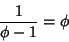(1)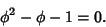(2)(3)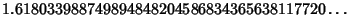(4)

(Sloane's A001622).A geometric definition can be given in terms of the above figure. Let the ratio. The Numerator and Denominator can then be taken asandwithout loss of generality. Now define the position of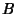by(5)

Plugging in gives(6)

or(7)

which can be solved using the Quadratic Equation to obtain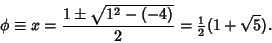(8)is the most'' Irrational number because it has a Continued Fraction representation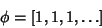(9)

(Sloane's A000012). Another infinite representation in terms of a Continued Square Root is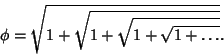(10)

Ramanujangave the curious Continued Fraction identities(11)(12)

(Ramanathan 1984).

The legs of a Golden Triangle are in a golden ratio to its base. In fact, this was the method used by Pythagoras to construct. Euclid used the following construction.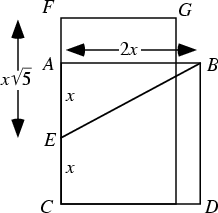Draw the Square, call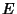the Midpoint of, so that. Now draw the segment, which has length(13)

and construct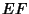with this length. Now construct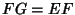, then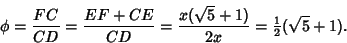(14)

The ratio of the Circumradius to the length of the side of a Decagon is also,(15)

Similarly, the legs of a Golden Triangle (an Isosceles Triangle with a Vertex Angle of 36°) are in a Golden Ratio to the base. Bisecting a Gaullist Cross also gives a golden ratio (Gardner 1961, p. 102).In the figure above, three Triangles can be Inscribed in the Rectangleof arbitrary aspect ratio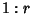such that the three Right Triangles have equal areas by dividingandin the golden ratio. Then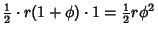(16)(17)(18)

which are all equal.

The golden ratio also satisfies the Recurrence Relation(19)

Takinggives(20)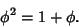(21)

But this is the definition equation for(when the root with the plus sign is used). Squaring gives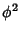(22)(23)

and so on.

For the difference equations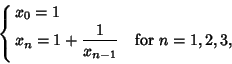(24)is also given by(25)(26)

whereis theth Fibonacci Number.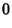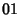(27)(28)

gives(29)

giving rise to the sequence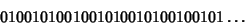(30)

(Sloane's A003849). Here, the zeros occur at positions 1, 3, 4, 6, 8, 9, 11, 12, ... (Sloane's A000201), and the ones occur at positions 2, 5, 7, 10, 13, 15, 18, ... (Sloane's A001950). These are complementary Beatty Sequences generated byand. The sequence also has many connections with the Fibonacci Numbers.

Salem showed that the set of Pisot-Vijayaraghavan Constants is closed, withthe smallest accumulation point of the set (Le Lionnais 1983).

See also Beraha Constants, Decagon, Five Disks Problem, Golden Ratio Conjugate, Golden Triangle, Icosidodecahedron, Noble Number, Pentagon, Pentagram, Phi Number System, Secant Method

References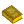Golden Ratio

Boyer, C. B. History of Mathematics. New York: Wiley, p. 56, 1968.

Coxeter, H. S. M. The Golden Section, Phyllotaxis, and Wythoff's Game.'' Scripta Mathematica 19, 135-143, 1953.

Dixon, R. Mathographics. New York: Dover, pp. 30-31 and 50, 1991.

Finch, S. Favorite Mathematical Constants.'' http://www.mathsoft.com/asolve/constant/cntfrc/cntfrc.html

Finch, S. Favorite Mathematical Constants.'' http://www.mathsoft.com/asolve/constant/gold/gold.html

Gardner, M. Phi: The Golden Ratio.'' Ch. 8 in The Second Scientific American Book of Mathematical Puzzles & Diversions, A New Selection. New York: Simon and Schuster, 1961.

Gardner, M. Notes on a Fringe-Watcher: The Cult of the Golden Ratio.'' Skeptical Inquirer 18, 243-247, 1994.

Herz-Fischler, R. A Mathematical History of the Golden Number. New York: Dover, 1998.

Huntley, H. E. The Divine Proportion. New York: Dover, 1970.

Knott, R. Fibonacci Numbers and the Golden Section.'' http://www.mcs.surrey.ac.uk/Personal/R.Knott/Fibonacci/fib.html.

Le Lionnais, F. Les nombres remarquables. Paris: Hermann, p. 40, 1983.

Markowsky, G. Misconceptions About the Golden Ratio.'' College Math. J. 23, 2-19, 1992.

Ogilvy, C. S. Excursions in Geometry. New York: Dover, pp. 122-134, 1990.

Pappas, T. Anatomy & the Golden Section.'' The Joy of Mathematics. San Carlos, CA: Wide World Publ./Tetra, pp. 32-33, 1989.

Ramanathan, K. G. On Ramanujan's Continued Fraction.'' Acta. Arith. 43, 209-226, 1984.

Sloane, N. J. A. Sequences A003849, A000012/M0003, A000201/M2322, A001622/M4046, A001950/M1332 in An On-Line Version of the Encyclopedia of Integer Sequences.'' http://www.research.att.com/~njas/sequences/eisonline.html and Sloane, N. J. A. and Plouffe, S. The Encyclopedia of Integer Sequences. San Diego: Academic Press, 1995.